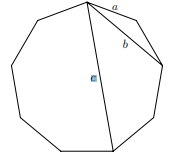## Thursday 27 October 2022

### Problems From the Land Down Under, and some moreLooking through the Gazette of the Australian Mathematical Society, and found their puzzle corner (July 2009 , so the exponents in the first problem are explained) ... really nice problems. I think I have this one, but I didn't prove it....

Digital deduction The numbers 2^2009 and 5^2009 are written on a piece of paper in decimal notation. How many digits are on this piece of paper? And this one has me puzzled (which is why they call them puzzles, I guess)..

Piles of stones There are 25 stones sitting in a pile next to a blackboard. You are allowed to take a pile and divide it into two smaller piles of size a and b, but then you must write the number a×b on the blackboard. You continue to do this until you are left with 25 piles, each with one stone. What is the maximum possible sum of the numbers written on the blackboard? Anyone know how to a) prove the first, or b) solve the second...

Do let me know....mostly down to chewing my pencil tips now....

Spoiler (I think) x x x x x x x
OK, for number one I went back to that old Polya-ism, "If there is a problem you can't solve, find a smaller problem you can solve."  Instead of 2010 I put in 1.  Well 2^1 has one digit and 5^1 has 1 digit so the answer is 2.  Repeating this with more numbers it seemed the solution was always n+1 digits for any exponent n.
Sue VanHattum gave a nice approach using base ten logarithms,
digits in 2^n = ceiling(log(2^n))
digits in 5^n = ceiling(log(5^n))
adding gives n+1, so we have n+1 digits.

OK, I think the total for the 25 stones will always be 300... I tried it about three different ways and they all came out the same... hmmmm... In fact, if we look at some smaller numbers for a guide, it seems that for any n, the sum of the products by this process will lead to $\binom{n}{2}$... now why is that? Anyone, Anyone??? Bueller?
Well I was right on that one, it seems, but the real understanding came when master problem solver, Joshua Zucker, explained, "The second problem I have seen many times in books as a strong induction exercise, but ... WHY does it come out the triangular numbers?
Well, the triangular numbers are the solution to the handshake problem.
When all the pebbles are in one pile, let them all shake hands.
At each splitting step, the number of points you score is equal to the number of handshakes you destroy.
At the end, you have all the pebbles in their own individual pile, so there are no more handshakes possible - they have all been destroyed.
Hence the score is equal to the initial number of handshakes.

For example, if you start with five stones/handshakes, there are 10 handshakes(edges) connecting the five points.

If you break away a group of two, (say V1 and V2) you break the connection between each of these two in one group and the three in the second group, or six handshakes, leaving four edges (handshakes) One between the set of two, and three in the triangle of V3, V4, V5.
------------------------

Just for a kick, I picked out a couple of newer ones for you to try.  Enjoy and share your solutions:

For the geometry lovers, try this one.

In a regular nonagon, prove that the length difference between the longest diagonal and the shortest diagonal is equal to the side length. In other words, prove c−b = a in the diagram below.*Australian Mathematical Soc. Gazette
And here is one for that I think is an excellent problem for younger students to intuit a wonderful mix of problems solving ideas.  "Let S be a set of 10 distinct positive integers no more than 100. Prove that S contains two disjoint non-empty subsets which have the same sum."

I will come back in awhile and address possible approaches to each, (If I can solve them).